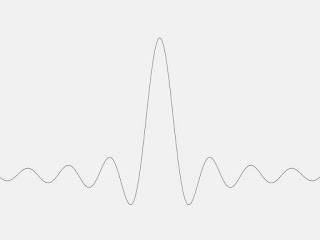## Friday, July 27, 2018

### Extrema of the Sinc Function

Intermediate Physics for Medicine and Biology: Extrema of the Sinc Function In Intermediate Physics for Medicine and Biology, Russ Hobbie and I write
The function sin(x)/x has its maximum value of 1 at x = 0. It is also called the sinc(x) function.
Sinc(x) oscillates like sin(x), but its amplitude decays as 1/x. If sin(x) is zero then sinc(x) is also zero, except for the special point x = 0, where 0/0 becomes 1.A plot of the sinc function.Trigonometric Delights, by Eli Maor
In IPMB, Russ and I dont evaluate the values of x corresponding to local maximum and minimum values of sinc(x). Eli Maor examines the peak values of f(x) = sinc(x) in his book Trigonometric Delights. He writes
We now wish to locate the extreme points of f(x)—the points where it assumes its maximum or minimum values. And here a surprise is awaiting us. We know that the extreme points of g(x) = sinx occur at all odd multiples of π/2, that is, at x = (2n+1)π/2. So we might expect the same to be true for the extreme points of f(x) = (sinx)/x. This, however, is not the case. To find the extreme point, we differentiate f(x) using the quotient rule and equate the result to zero:

f’(x) = (x cosx – sinx)/x2 = 0.         (1)

Now if a ratio is equal to zero, then the numerator itself must equal to zero, so we have x cosx – sinx = 0, from which we get

tan x = x.                                         (2)

Equation (2) cannot be solved by a closed formula in the same manner as, say, a quadratic equation can; it is a transcendental equation whose roots can be found graphically as the points of intersection of the graphs of y = x and y = tan x.A plot of y=tanx versus x and y=x versus x.

The extreme values are at x = 0, 4.49 = 1.43π, 7.73 = 2.46π, etc. As x becomes large, the roots approach (2n+1)π/2.

Eli Maor is a rare breed: a writer of mathematics. Russ and I cite his wonderful book e, The Story of a Number in Chapter 2 of IPMB. I also enjoyed The Pythagorean Theorem: A 4,000-year History. Maor has written many books about math and science. His most recent came out in May: Music by the Numbers--From Pythagoras to Schoenberg. I put it on my summer reading list.